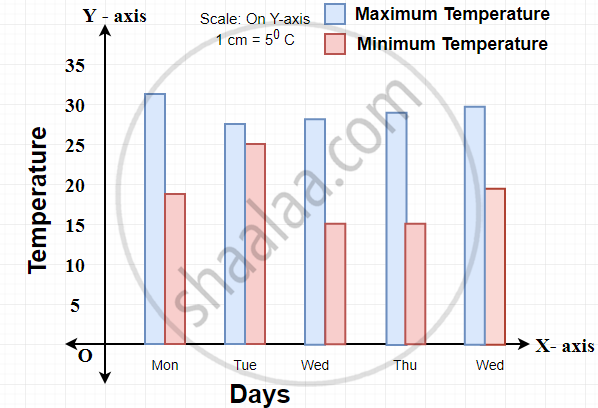# Interpretation of a Double Bar Graph

#### definition

Interpretation of a double bar graph: Interpretation of a double bar graph means the action of explaining the meaning of the double bar diagram.

# Interpretation of the double bar graph:

Interpretation of a double bar graph means the action of explaining the meaning of the double bar diagram.

1) The minimum and maximum temperature in Pune for five days is given. Read the joint bar graph and answer the questions below.1. What data is shown on X-axis?

2. What data is shown on Y-axis?

3. Which day had the highest temperature?

4. On which day is the minimum temperature the highest?

Solution:

1. Days are shown on X-axis.

2. Temperature is shown on Y-axis.

3. Monday had the highest temperature.

4. Tuesday had the minimum temperature the highest.

If you would like to contribute notes or other learning material, please submit them using the button below.

### Shaalaa.com

Interpreting a double bar graph [00:02:44]
S
0%